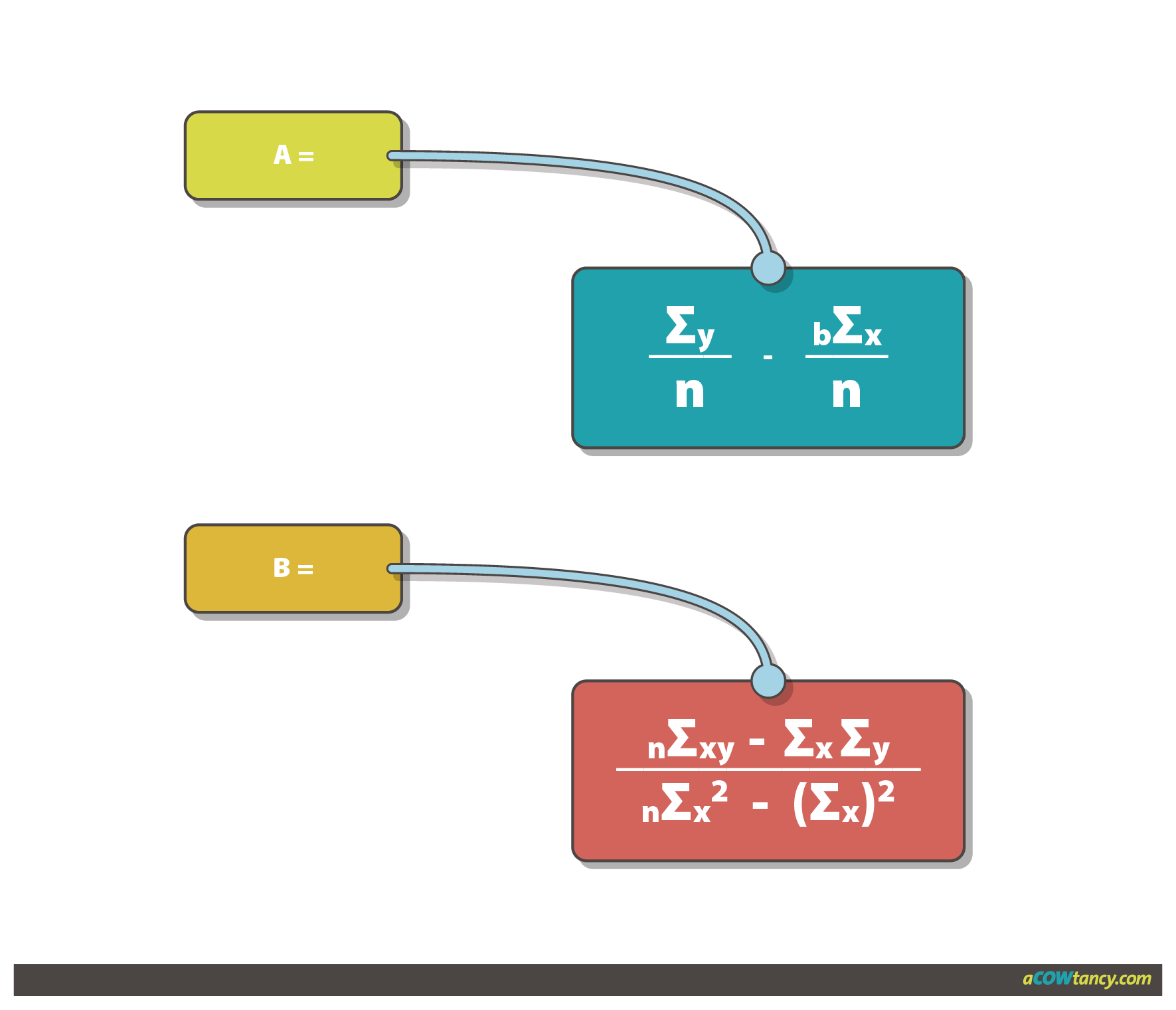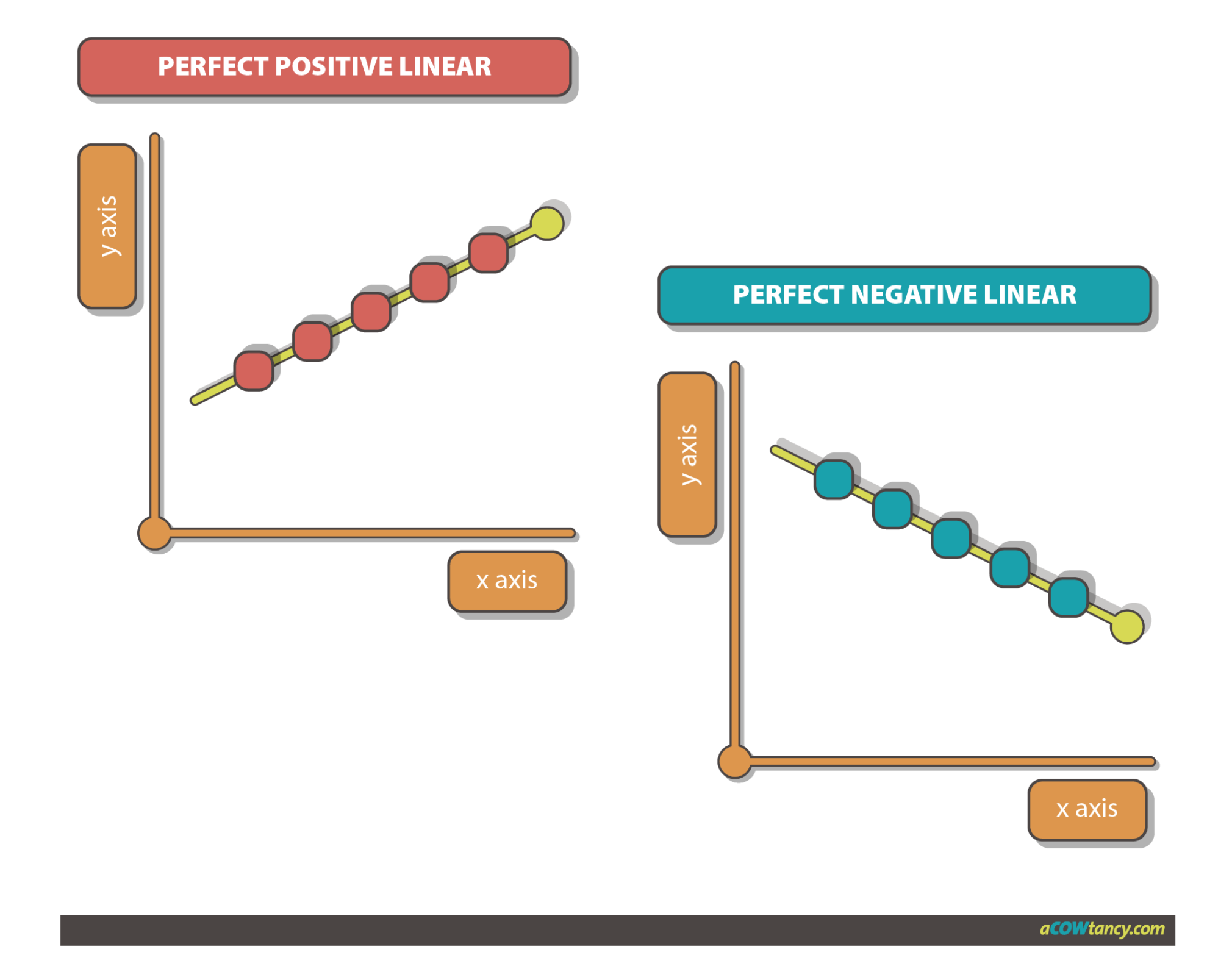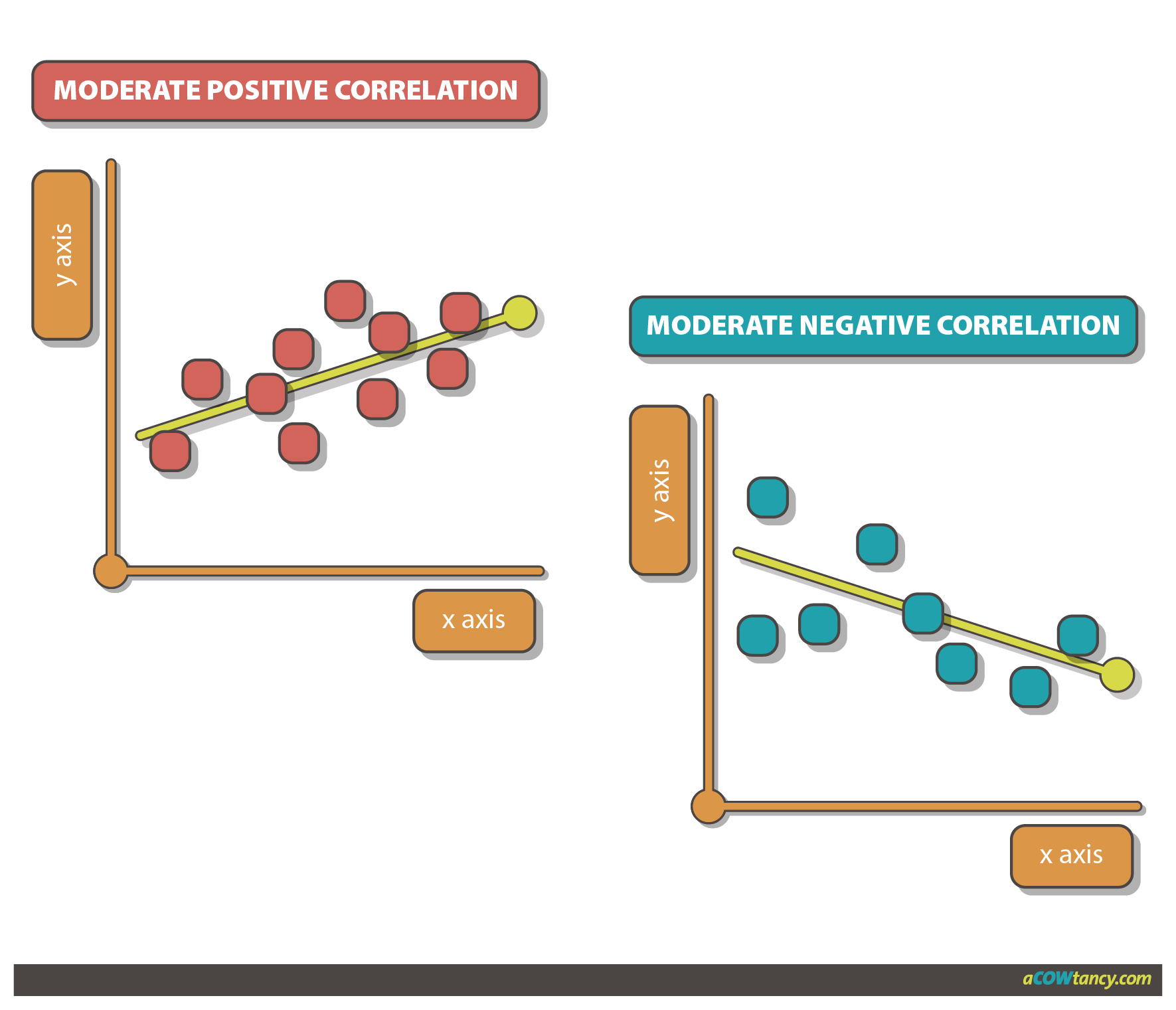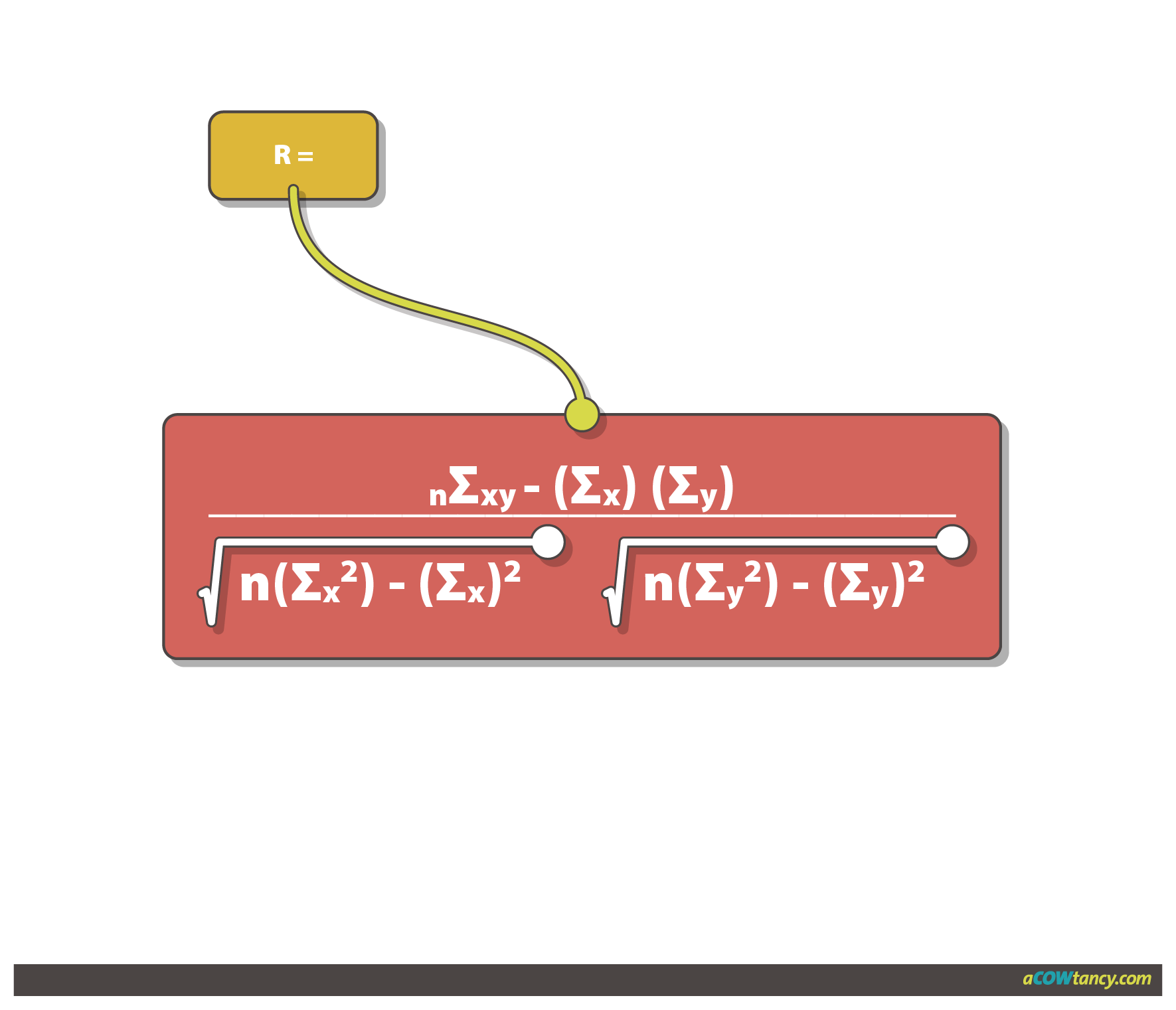MAF2
Syllabus B. Data Analysis And Statistical Techniques B2. Forecasting techniques

# B2e. Analysis of cost data

### Syllabus B2e)

Analysis of cost data.

(i) Explain the concept of correlation coefficient and coefficient of determination.
(ii) Calculate and interpret correlation coefficient and coefficient of determination.
(iii) Establish a linear function using regression analysis and interpret the results.

### Linear Regression Analysis

Linear regression analysis is based on working out an equation for the line of best fit.

The line of best fit will be of the form
y = a + bx
where
y is the value of the dependent variable (on vertical axis)
a is the intercept - fixed costs
b is the slope of the line - variable costs
x is the value of the independent variable (on horizontal axis)

i. How do we calculate 'a' and 'b'?#### How do we calculate ‘a’ and ‘b’?

‘a’ is the fixed cost per period
‘b’ is the variable cost per unit
‘x’ is the activity level (independent variable)’
‘y’ is the total cost = fixed cost + variable cost
‘n’ is the sample size – number of pairs of data

These formulae are given in the exam. Remember always start working ‘b’, then move to ‘a’.

#### Correlation

Correlation measures the strength of the relationship between two variables.

One way of measuring ‘how correlated’ two variables are, is by drawing the ‘line of best fit’ on a scatter graph.  When correlation is strong, the estimated line of best fit should be more reliable.

Another way of measuring ‘how correlated’ two variables are, is to calculate a correlation coefficient, r.

### Different degrees of correlation#### The correlation coefficient (r)

The correlation coefficient measures the strength of a linear relationship between two variables.  It can only take on values between -1 and +1.

r = +1 indicates perfect positive correlation

r = 0 indicates no correlation

r = -1 indicates perfect negative correlation

The correlation coefficient is calculated as follows

This formula is also given in the exam#### Coefficient of determination (r2)

The coefficient of determination is the square of the correlation coefficient.

It measures how much of the variation in the dependent variable is ‘explained’ by the variation of the independent variable.

The value of r2 can be between 0 and 1.

For example, if r = 0.97, r2 = 0.94 or 94%.

94% of the variation in the dependent variable (y) is due to variations in the independent variable (x). 6% of the variation is due to random fluctuations.

Therefore, there is high correlation between the two variables.